Next: 7.3 Null Sequences Up: 7. Complex Sequences Previous: 7.1 Some Examples.   Index

# 7.2 Convergence

7.6   Definition (Convergent sequence.) Let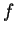be a complex sequence, and let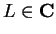. We will sayconverges to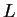and write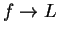if for every disc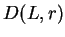there is a number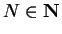such thatWe sayconverges if there is somesuch that. We saydiverges if and only ifdoes not converge.

It appears from figure a 7.1 that for every disc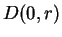centered at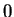the terms of the sequence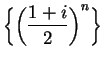eventually get into; i.e., it appears that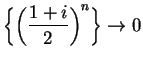. Similarly, it appears that.

From figure b, it appears that there are numbers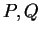such that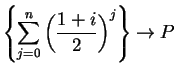, and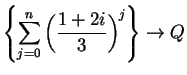. You should be able to put your finger on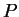and, and maybe to guess what their exact values are. We will return to these examples later.

Let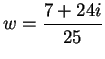. The figure below represents the sequence. It appears from the figure that there is no numbersuch that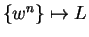. The following theorem shows that this is the case. (Note that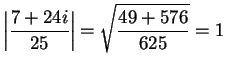.)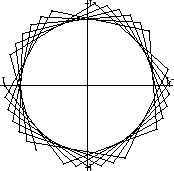7.7   Theorem. Let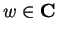satisfy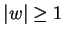and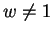. Thendiverges.

Proof: Suppose thatand. Then for all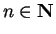,(7.8)

Now suppose, to get a contradiction, that there is a numbersuch that. Then corresponding to the disc, there is a numbersuch thatIn particular,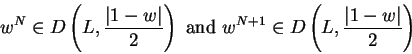so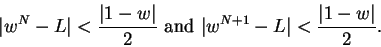By the triangle inequality,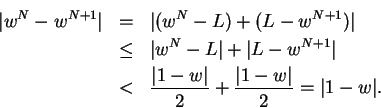Combining this result with (7.8), we getso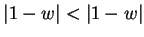. This contradiction shows thatdiverges.We can also show that constant sequences converge.

7.9   Theorem. Let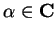. Then the constant sequence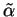converges to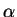.

Proof: Let. Letbe a disc centered at. Then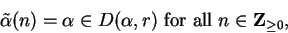Hence,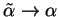.For purposes of calculation it is sometimes useful to rephrase the definition of convergence. Since the discis determined by its radius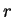, and for all,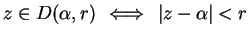, we can reformulate definition 7.6 as

7.10   Definition (Convergence.) Letbe a sequence in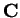, and let. Thenif and only if for every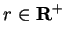there is somesuch that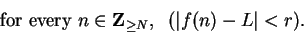Next: 7.3 Null Sequences Up: 7. Complex Sequences Previous: 7.1 Some Examples.   Index